# CIP Filter

This page provides user documentation for the filter functions of the CIP package

One can found additional information on the function parameters (type, optional/required, positional/named) on the parameters documentation page.

To access another category of CIP functions: Format, Filter, Math, Segment, Assess, Experimental.

# gauss

Description
gauss creates a gaussian blurred image. it convolves the image with a gaussian weighted window. Gaussian blurring is commonly used for image denoising as it smoothes out small details.

Signature

`outputImage = cip.gauss( inputImage* , radius* , boundary , pixelSize)`

Input

inputImage* : the image to process
radius* : a scalar or list of scalar representing the standard deviation ofthe gaussian kernel standard deviation along the image axis
boundary : a string in {‘min’, ‘max’, ‘same’, ‘zero’, ‘mirror’,’periodic’}, default is mirror
pixelSize : a scalar or list of scalar along image dimension. Default is 1.

Output

outputImage: the processed image. it always as the same size as the input image.

Example

`img2 = cip.gauss( img1 , 5 )`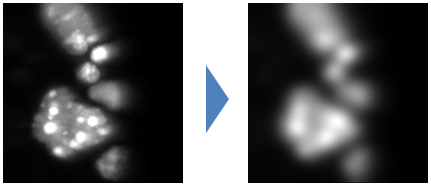Implementation
CIP gauss implementation wraps the gauss ops, itself relying on the imglib2 gauss3 implementation.

# erode

Description
Erosion shrinks the region in an image by a certain radius. It works both with binary and graylevel images. This effect is obtained by replacing each pixel value by the minimum value found in a window surrounding that pixel.

Signature

`outputImage = cip.erode( inputImage*, radius*, shape, boundary, pixelSize)`

Input

inputImage* : the image process
radius* : a integer or list of integer representing the half size of thefilter window along the image axis.
shape : a string in {‘rectangle’, ‘disk’} describing the shape of thewindow used for the processing.
boundary : a string in {‘min’, ‘max’, ‘same’, ‘mirror’, ‘periodic’},default is mirror
pixelSize : a scalar or list of scalar along image dimension. Default is 1.

Output

outputImage: the processed image.

Example

`img2 = cip.erode( img1 , 2 )`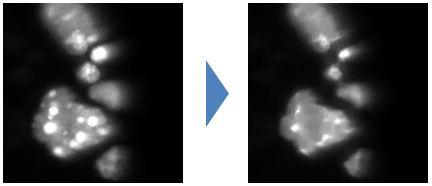Implementation
CIP function wraps the imglib2 Erosion class from the morphology package.

# dilate

Description
this filter dilate the region in an image by a certain radius. It works both with binary and graylevel images. This effect is obtained by replacing each pixel value by the maximum value found in a window surrounding that pixel.

Signature

`outputImage = cip.dilate( inputImage* , radius*, shape, boundary, pixelSize)`

Input

inputImage* : the image process
radius* : a integer or list of integer representing the half size of thefilter window along the image axis.
shape : a string in {‘rectangle’, ‘disk’} describing the shape of thewindow used for the processing.
boundary : a string in {‘min’, ‘max’, ‘same’, ‘mirror’, ‘periodic’},default is mirror
pixelSize : a scalar or list of scalar along image dimension. Default is 1.

Output

outputImage: the processed image.

Example

`img2 = cip.dilate( img1 , 2 )`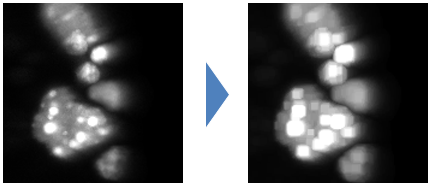Implementation

CIP function wraps the imglib2 Dilation class from the morphology package.

# opening

Description
This filter performs an erosion followed by a dilation. It erases small and thin objects.

Signature
`outputImage = cip.opening( inputImage* , radius* , shape, boundary, pixelSize)`

Input

inputImage* : the image process
radius* : a integer or list of integer representing the half size of thefilter window along the image axis.
shape : a string in {‘rectangle’, ‘disk’} describing the shape of thewindow used for the processing.
boundary : a string in {‘min’, ‘max’, ‘same’, ‘mirror’, ‘periodic’},default is mirror
pixelSize : a scalar or list of scalar along image dimension. Default is 1.

Output

outputImage: the processed image.

Example

`img2 = cip.opening( img1 , 5 )`Implementation
CIP function wraps the imglib2 Opening class from the morphology package.

# closing

Description
This filter performs a dilation followed by an erosion. It closes small holes and thin gaps between or inside objects.

Signature

`outputImage = cip.closing( inputImage* , radius* , shape, boundary, pixelSize)`

Input

inputImage* : the image process
radius* : a integer or list of integer representing the half size of thefilter window along the image axis.
shape : a string in {‘rectangle’, ‘disk’} describing the shape of thewindow used for the processing.
boundary : a string in {‘min’, ‘max’, ‘same’, ‘mirror’, ‘periodic’},default is mirror
pixelSize : a scalar or list of scalar along image dimension. Default is 1.

Output

outputImage: the processed image.

Example

`img2 = cip.closing( img1 , 16, 'disk' )`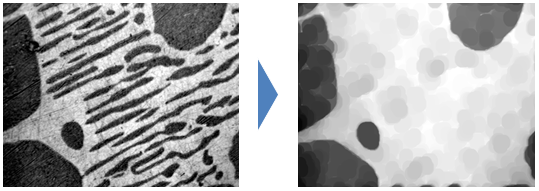Implementation
The implementation successively applies CIP erosion and dilation functions.

# tophat

Description
This filter subtract an opening of the input image to the input image. It removes object larger than the user selected radius in image while keeping smaller scale details.

Signature

`outputImage = cip.closing(inputImage*, radius*, shape, boundary, pixelSize)`

Input

inputImage* : the image process
radius* : a integer or list of integer representing the half size of thefilter window along the image axis.
shape : a string in {‘rectangle’, ‘disk’} describing the shape of thewindow used for the processing.
boundary : a string in {‘min’, ‘max’, ‘same’, ‘mirror’, ‘periodic’},default is mirror
pixelSize : a scalar or list of scalar along image dimension. Default is 1.

Output

outputImage: the processed image.

Example

`img2 = cip.tophat( img1 , 5, 'disk' )`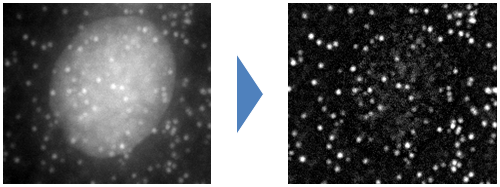Implementation
The function relies on ops for subtraction and CIP for the opening.

# distance

Description
this function create an image where each pixel value correspond between the distance of that pixel to the closest background object in the input image

Signature

`outputImage = cip.distance(inputImage*, threshold, pixelSize)`

Input

inputImage* : the image process
threshold : if the input image is not binary that value can be used tothreshold the image. if the input image is binary that value is ignored
pixelSize : a scalar or a list of scalar representing pixel size along imagedimension. Default is 1.

Output

outputImage: the processed image.

Example

`img2 = cip.distance( img1 , 500 )` or `img2 = cip.distance( img1 , 'threshold', 500 )`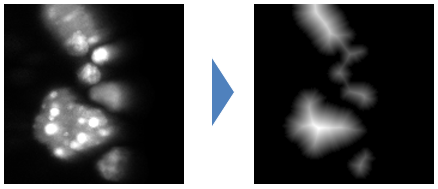`img2 = cip.distance( img1 )` will build the distance map for a binary image.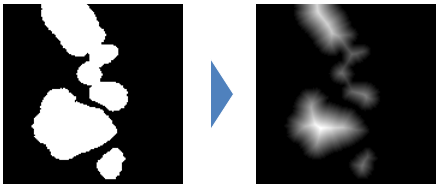Implementation
The function relies on ops distance function implementation.

# median

Description
This filter is used to denoise image. It is well suited to remove impulse noise.Compare to the gaussian filter it preserve edges better but has higher computationnal cost. Its basic principle is to replace a pixel value with the median value in a window surrounding that pixel.

Signature

`outputImage = cip.median(inputImage*, radius*, shape, boundary, pixelSize)`

Input

inputImage* : the image process
radius* : a integer or list of integer representing the half size of thefilter window along the image axis.
shape : a string in {‘rectangle’, ‘disk’} describing the shape of thewindow used for the processing.
boundary : a string in {‘min’, ‘max’, ‘same’, ‘mirror’, ‘periodic’},default is mirror
pixelSize : a scalar or list of scalar along image dimension. Default is 1.

Output

outputImage: the processed image.

Example

`img2 = cip.median( img1 , 5 )`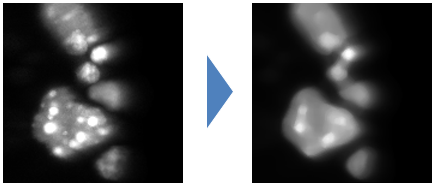Implementation
The function relies on ops that implement a brute force approach of the median filtering. It would be possible to implement more efficient approach using histogram and cord decomposition such ImageJ 1.x implementation for 2D image.

# invert

Description
This function invert the gray value of the input image such that each pixel value I is replaced by max+min-I , where are min (resp max) are the minimum (resp maximum) intensity in the input image.

Signature

`outputImage = cip.invert(inputImage*)`

Input

inputImage* : the image to process

Output

outputImage: the processed image.

Example

`img2 = cip.invert( img1 )`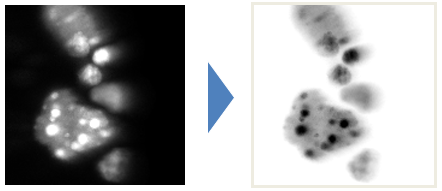Implementation
The function implementation uses ops map function